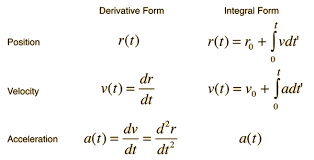Education

# Choosing the Right Sensing Method for Velocity Based Training: A Comparative Analysis

In this blog article, we’ll be comparing different sensing methods and their strengths, weaknesses, and why we choose to use the tech in RepOne Sensor.

The following sensing methods revolve around Velocity Based Training (VBT). If you don’t know already, VBT is a training methodology that uses technology to optimize performance in strength training. By using VBT, athletes and coaches can determine the appropriate load and intensity for each exercise, ensuring optimal training outcomes and reducing the risk of injury.

Various sensing methods can be used in VBT devices to acquire velocity data, including Linear Position Transducers (LPT), Accelerometers, Camera-based systems, and Laser-based units. Each device has its advantages and limitations in terms of accuracy, ease of use, and cost.

## Warning - math incoming

Before we begin, a short (and painless, I promise) math lesson is in order. If you’re in STEM, you may remember the following equations from pre-algebra or physics.Velocity can be calculated as the derivative of position, and acceleration as the derivative of velocity. The same calculation can be made in reverse, where velocity is the integral of acceleration, and position is the integral of velocity. OK, math lesson over.

The above information is important because each sensing type specializes in a specific measurement domain. LPT’s and cameras directly measure position. Lasers directly measure either position or velocity (or both), and accelerometers measure, you guessed it, acceleration.

At this point, given the equations above, you may think that if you measure one metric you can easily derive the other two. On paper, given an accurate enough sensor, each of these sensor types should provide equally valid measurements of position, velocity, and acceleration. The issue arises when you move from paper to practical, and measurement error is introduced.

Every measurement has an associated error. The thermometer in your oven is likely only accurate to within tens of degrees, notwithstanding measurement issues from placing your food on the wrong rack, which is a topic for another blog post.

Measurement error becomes relevant when you do the math via derivative vs integral. In other words, if you directly measure position and try to calculate velocity, vs directly measuring acceleration and trying to calculate velocity.

A discrete derivative (a derivative done in a microcontroller rather than on a piece of paper) to calculate velocity from position is straightforward. It’s the more current position measurement, less the previous position measurement, divided by the time measured between each.

V=P2-P1t

You’ll notice you do a subtraction, then a division. Let’s see what happens when we introduce some error.

Ve=(P2+e2)-(P1+e1)(t+e3)

Ve=(P2-P1)t+e3+(e2-e1)t+e3

In the above scenario, you’re doing two things with your error that are very convenient. First, you’re subtracting your errors. That doesn’t mean the errors will eliminate each other. They may, but that’s statistically random and won’t help with measurement validity. But it’s much better than adding or even multiplying your errors. Then, you divide by the error in your time measurement. Again, division is better than multiplication. What you end up with is an error scenario that definitely could be worse.

Let’s investigate the reverse direction, calculating velocity from acceleration measurement, and then adding in our error.

V2=V1+(A2-A1)*t

With error

V2+e=V1+e+(A2-A1)*(t+e)+(e2-e1)*(t+e))

You’ll notice that although we do have a couple of error figures subtracting, we’re also multiplying our errors and adding them to the result of the previous calculation, which includes its own set of errors.

This phenomenon is known as sensor drift, and is such a difficult problem to solve that even the US Military had to give up IMU-only sensing for missile targeting purposes. That doesn’t mean accelerometers are useless in exercise, though, because the short duration of a single repetition allows algorithms to ‘reset’ drift after each rep.

## Diving into each method

Linear Position Transducers (LPT) are currently considered the gold standard for measuring bar speed and velocity in VBT. These devices use a tether that connects the bar to a sensor, allowing for highly precise measurements of displacement and velocity. They typically do this by rotating a measurement target, either magnetic, optical, inductive, or potentiometer-based, and counting ‘pulses’ that correspond to degrees of rotation. Calculating the rate of pulses yields a velocity reading.

LPTs can be highly accurate and reliable, with a measurement error of as little as  1%. They also have high sampling rates, which means that they can capture data at a high frequency, making them suitable for use in dynamic and explosive movements.

LPTs do well in applications that require a broad spectrum of metric since, as we detailed above, their measurements start at position and work their way up to acceleration-based metrics like Power and Force. They won’t measure force as well as something like a strain gauge, as seen in a force plate, but it’ll get the job done.